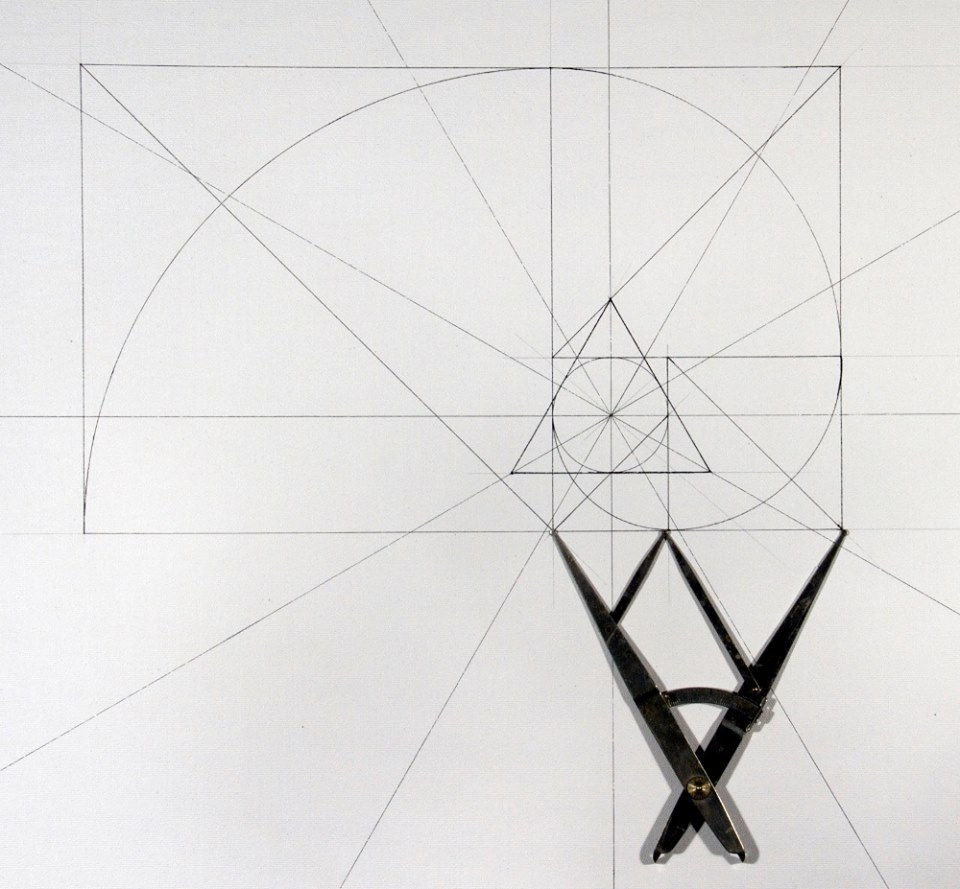top of page

# Fibonacci

The Fibonacci sequence is named after Italian mathematician Fibonacci. His 1202 book Liber Abaci introduced the sequence to Western European mathematics, although the sequence had been described earlier in Indian mathematics. By modern convention, the sequence begins either with F0 = 0 or with F1 = 1. The Liber Abaci began the sequence with F1 = 1.

Fibonacci numbers are closely related to Lucas numbers in that they are a complementary pair of Lucas sequences. They are intimately connected with the golden ratio; for example, the closest rational approximations to the ratio are 2/1, 3/2, 5/3, 8/5, ... Applications include computer algorithms such as the Fibonacci search technique and the Fibonacci heap data structure, and graphs called Fibonacci cubes used for interconnecting parallel and distributed systems. They also appear in biological settings, such as branching in trees, phyllotaxis (the arrangement of leaves on a stem), the fruit sprouts of a pineapple, the flowering of an artichoke, an uncurling fern and the arrangement of apine cone's bracts.Tags:

bottom of page